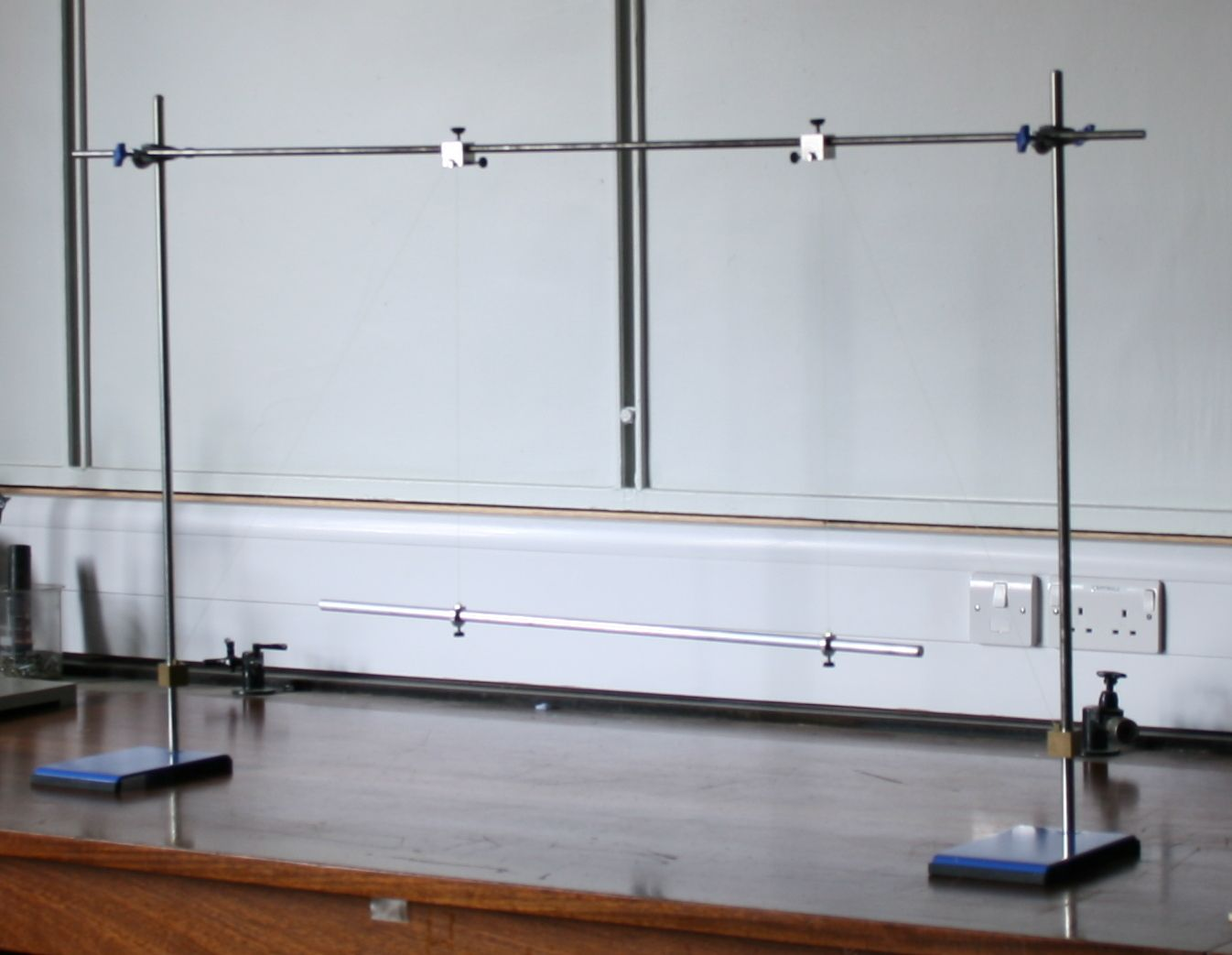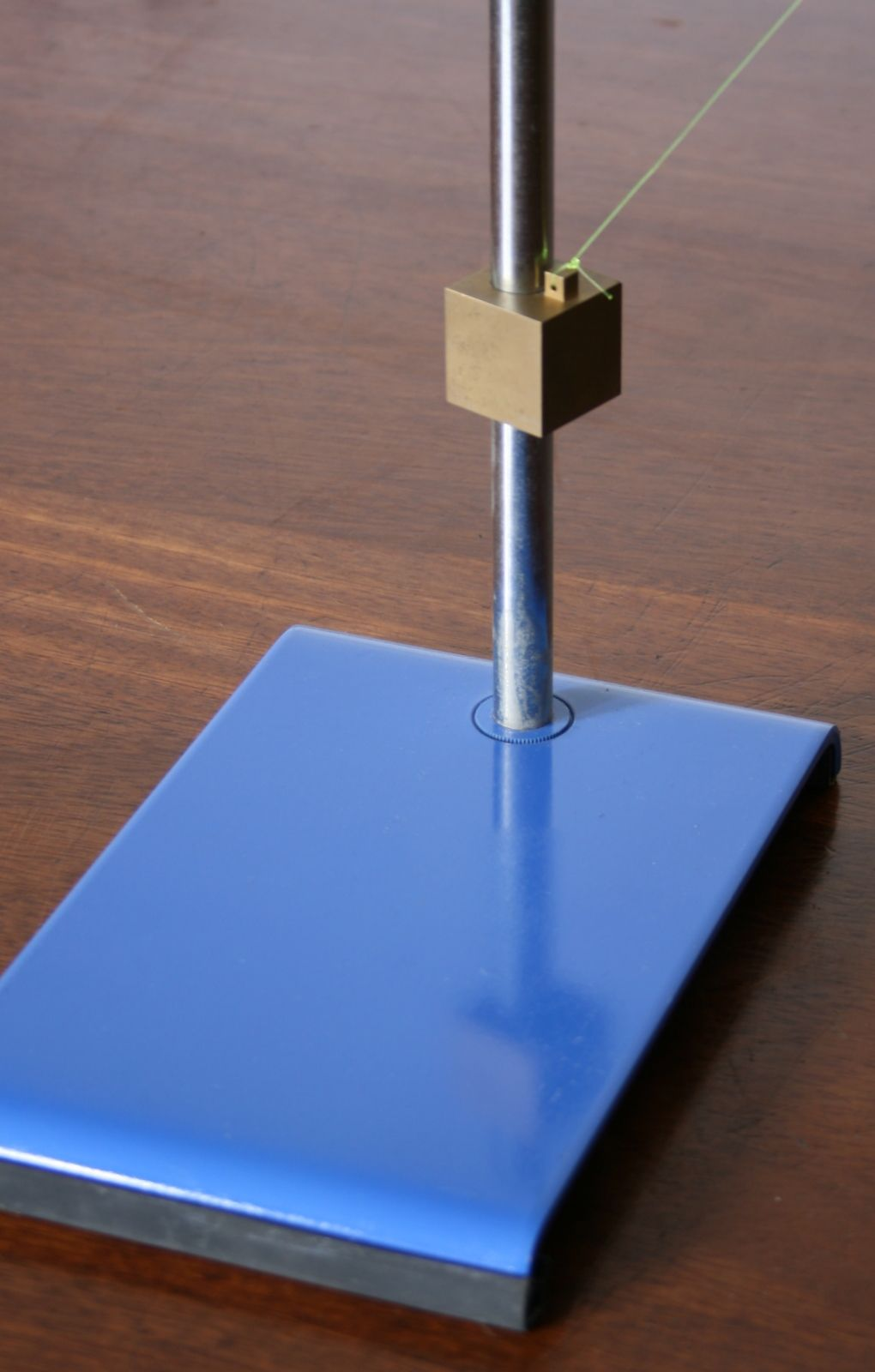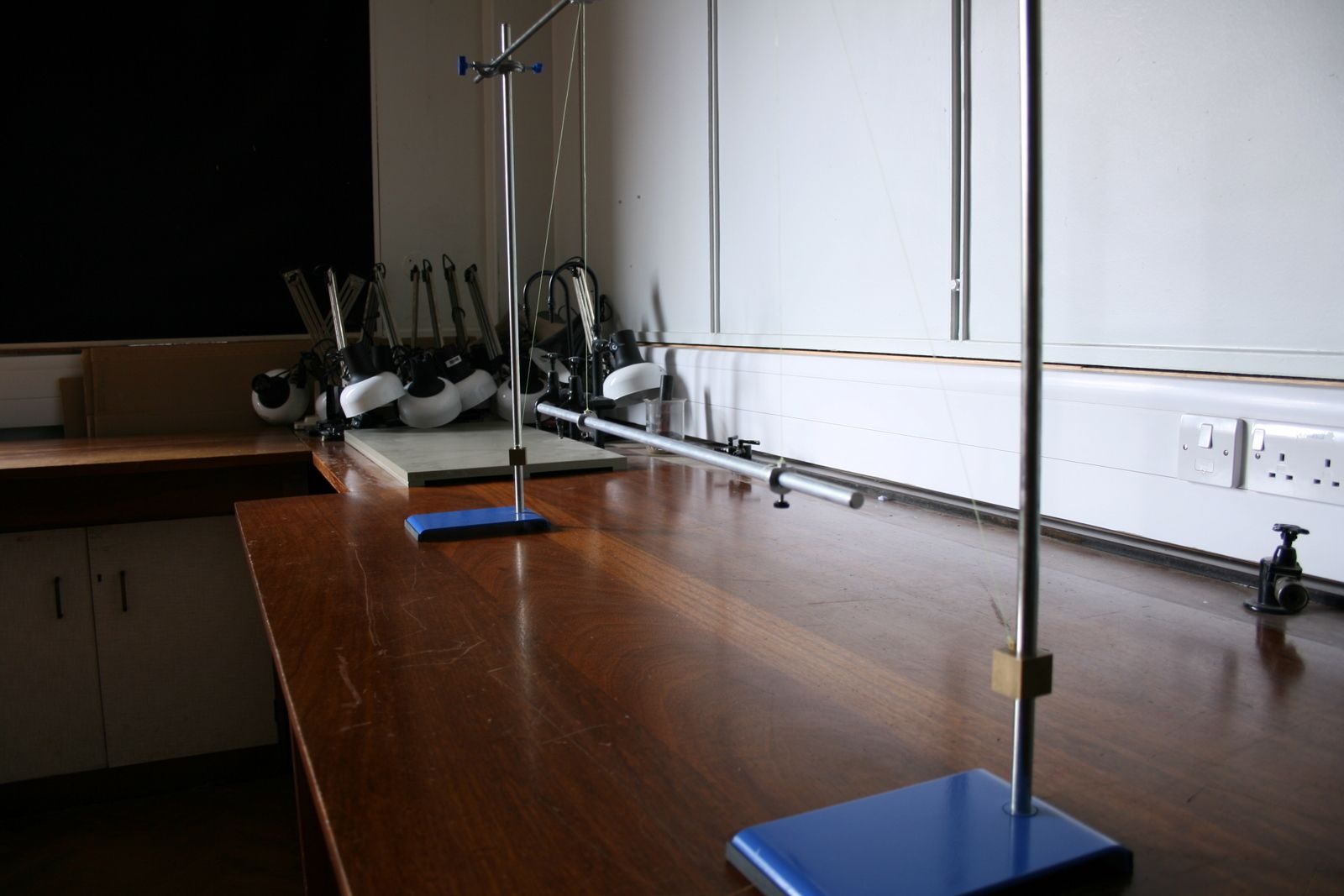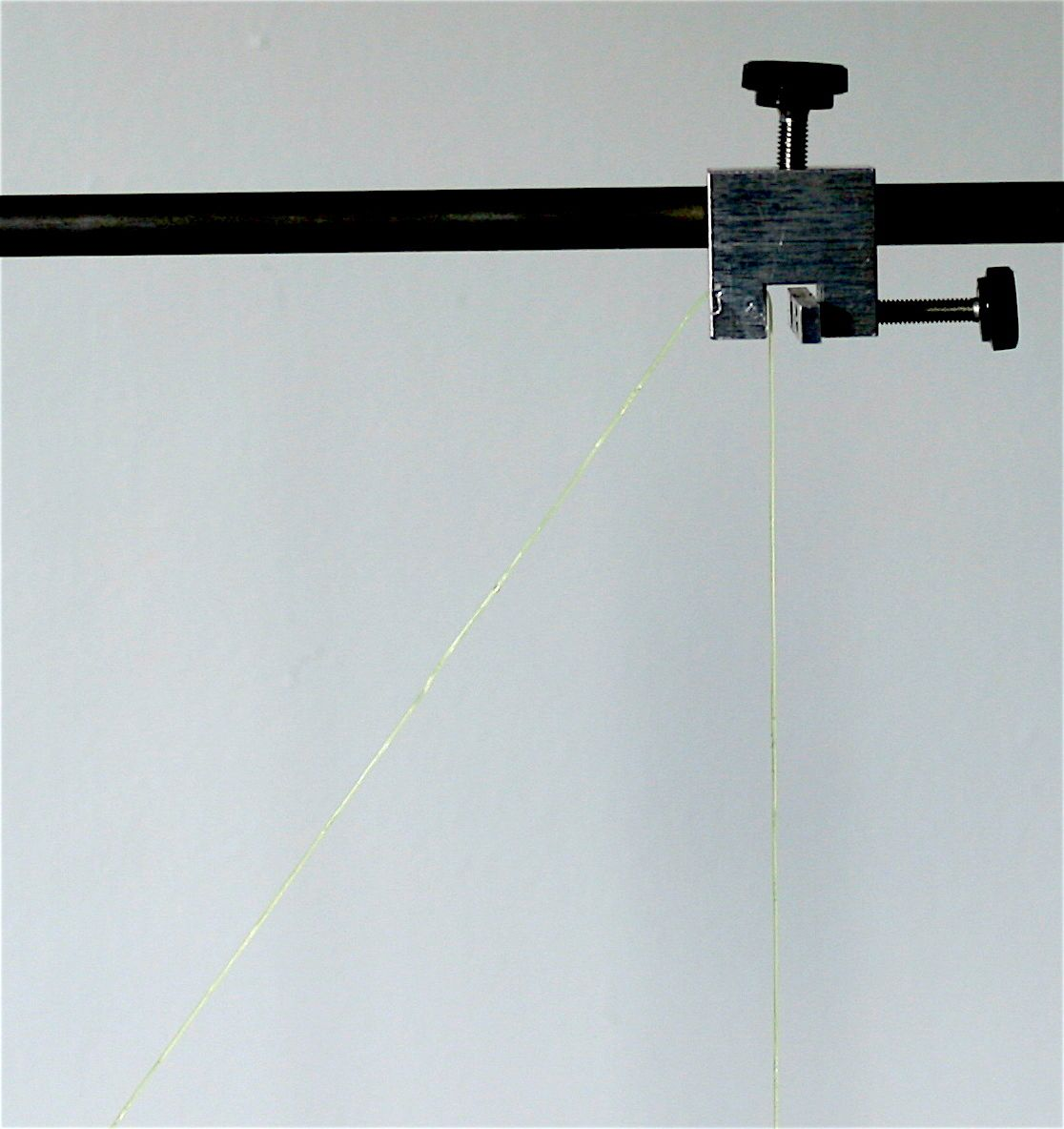Bifilar pendulum set-upTwine attached to the crossbarBifilar pendulum set-upTwine attached to upright

## Bifilar Pendulum

### Introduction

This practical explores the use of dimensional analysis in the formulation of an equation. You will set-up an equation for the period of oscillation of a complex pendulum as shown in figure 1. Dimensional analysis will be used to ensure that the equation is dimensionally correct and you will subsequently determine experimentally the exponents in the proposed equation. This is an idealised experiment, where your results will be improved by taking multiple, repeat measurements and applying a statistical analysis to the data.

### Script

The script for this experiment can be found in the lab script book or on DUO.